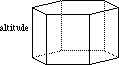index: click on a letter A B C D E F G H I J K L M N O P Q R S T U V W X Y Z A to Z index index: subject areas numbers & symbols sets, logic, proofs geometry algebra trigonometry advanced algebra & pre-calculus calculus advanced topics probability & statistics real world applications multimedia entrieswww.mathwords.com about mathwords website feedback

 Altitude of a Prism Height of a Prism The distance between the two bases of a prism. Formally, the shortest line segment between the (possibly extended) bases. Altitude also refers to the length of this segment.See also Oblique prism, right prism, volume, surface area